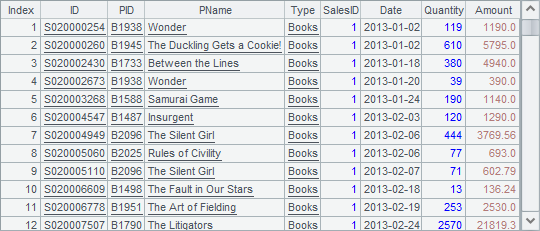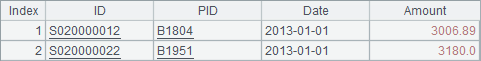# 大数据结果集的外存分组原理

 A B C 1 1000000 1000 =A1/B1 2 =file("BirthStateRecord") =create(ID,Birthday,State) =demo.query("select ABBR from STATES") 3 1994-1-1 0 =C2.(ABBR) 4 for C1 for B1 >B3=B3+1 5 =elapse(A3,-rand(10000)) 6 =C3(rand(C3.len())+1) 7 >B2.insert(0,B3,C5,C6) 8 >A2.export@ab(B2) 9 >B2.reset() 10 =A2.cursor@b() >A10.skip(50000) =A10.fetch(1000) 11 >A10.close()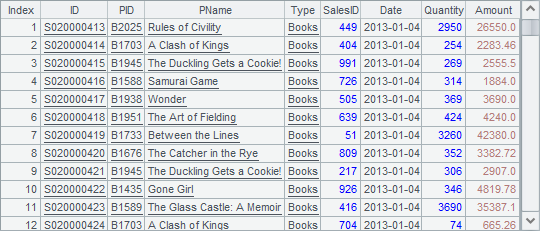A 1 =file("BirthStateRecord") 2 =A1.cursor@b() 3 =A2.groups(State;count(~):Count) 4 >A2.close()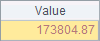A 1 =file("BirthStateRecord") 2 =A1.cursor@b() 3 =A2.groupx(Birthday;count(~):Count;1000) 4 =A3.fetch(1000) 5 >A2.close()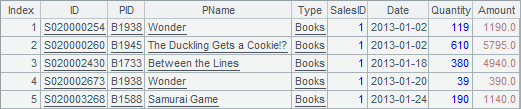A3中代码执行后，就会在临时文件目录下，生成外存文件，可以在临时文件目录下找到这些文件：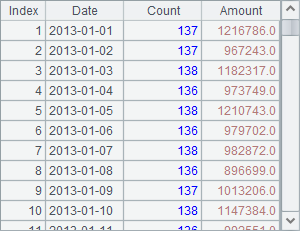A 1 =file("/temp/tmpdata1786998866046507792") 2 =A1.import@b() 3 =A2.count()

A2中读取到的数据如下：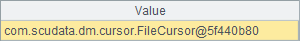A3中的数据如下：